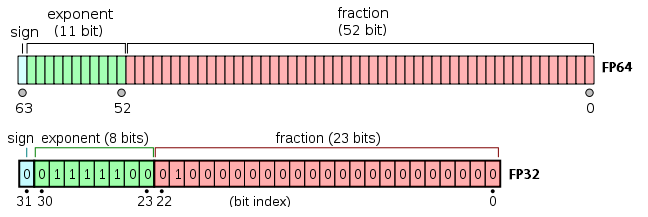# Single Precision vs Double Precision

Here you will learn about Single Precision vs Double Precision.

When talking about the numbers, amount of precision that can be reached using a representation technique has always been the major area of interest for the researchers. This curiosity to increase the precision and challenge the representation limits of numerical values, in computer science, lead to two major achievements – single precision and double precision.

### Single Precision

Single precision is the 32 bit representation of numerical values in computers. It is also known as binary32. Some languages, like JAVA, C++ use float to store these kinds of numerals. Some languages (Visual Basic) refer single numerals as single.

Single precision is most widely used because of its capability to represent wide range of numeral values, though it reduces the amount of precision achieved.

Single precision uses 32 bit to represent a floating point number.

First bit represent the sign of the number, negative or positive.

Next 8 bits are used to store the exponent of the number. This exponent can be signed 8-bit integer ranging from -127 – 128 of signed integer (0 to 255).

And the left 23 bits are used to represent the fraction part and are called fraction bits.

8 exponent bits provide us with the range and 23 bits provide us with the actual precision.

### Double Precision

Double precision is called binary64. Double precision uses 64 bits to represent a value.

First bit is used for the same purpose as in single point precision i.e., represents sign of the number.

Next 11 bits are used to denote exponent, which provide us with the range, and has 3 more bits than single precision, so it is used to represent a wider range of values.

Next 52 bits are used to represent the fractional part which is 29 bits more than binary32 bit representation scheme. So it has a greater precision than single precision.

Double floating point precision are used where high arithmetic precision is required and number like – 2/19 have to be used. Double point precision requires more memory as compared to single precision, hence are not useful when normal calculations are to be performed. This representation technique finds its use in the scientific calculations.Image Source

## Single Precision vs Double Precision

 Single Precision Double Precision Is binary32 bit representation scheme. Is binary64 bit representation scheme. Uses 32 bit memory to represent a value. Uses 64 bit memory to represent a value. Uses 1 bit to represent sign. Uses 1 bit to represent sign. Uses 8 bits to represent exponent. Uses 11 bits to represent exponent. Uses 23 bits to represent fractional part. Uses 52 bits to represent fractional part. Is widely used in games and programs requiring less precision and wide representation. Finds its use in the area to scientific calculations, where precision is all that matters and approximation is to be minimized.

Comment below if you queries related to difference between Single Precision and Double Precision.

### 2 thoughts on “Single Precision vs Double Precision”

1.Thank you for your good explanations.

2.great explanation. wish I had it in my inventory when dealing with describing details to team members when beta testing packet switching and interrupt code detection, , , back in the day